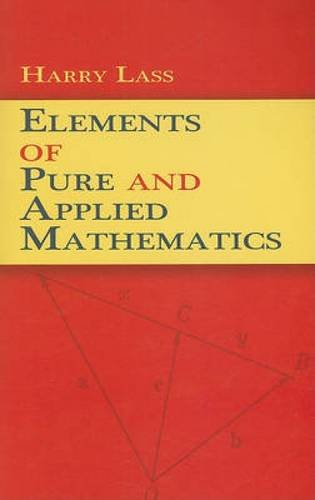# Elements of Pure and Applied Mathematics (Dover Books on Mathematics)Type
Book
Authors
ISBN 10
0486471861
ISBN 13
9780486471860
Category
Unknown  [ Browse Items ]
Edition
Reprint
Publication Year
2009
Publisher
Pages
512
Abstract

Description
Completely self-contained, this survey offers a single-volume exploration of all the important topics in pure and applied mathematics. Each chapter can be read independently of the others, and all subjects are unified by cross-references to the complete work. Every section concludes with review questions and references, and numerous worked-out examples appear throughout the text.Starting with discussions of determinants, linear equations, and matrices, the text advances to a review of the essentials of vector analysis. Subsequent chapters include explorations of tensor calculus, a rigorous treatment of complex variable theory, an undergraduate-level treatment of differential equations, and a consideration of orthogonal polynomials, Fourier series, and Fourier integrals appropriate for mathematicians, physicists, and engineers. Additional topics include the Stieltjes integral, the Laplace transform, the calculus of variations, group theory and algebraic equations, probability theory and statistics, and real variable theory. - from Amzon
Biblio Notes

Number of Copies